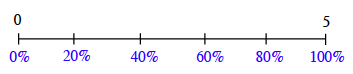### Home > CC1MN > Chapter 3 Unit 3A > Lesson CC1: 3.1.2 > Problem3-32

3-32.

At Cassie’s Cashew Shoppe, a sign says, “Today only: $20\%$ off anything.” Maribel realizes that she has a coupon for $\frac { 1 } { 5 }$ off the price of anything in the shop. Which discount should she use, the $20\%$-off deal or the $\frac { 1 } { 5 }$-off coupon? Does it matter? Explain.

$20\% \text{ is the same as }\frac{20}{100}.\text{ Can you use a percent ruler or number line to represent this?}$

$\text{Using the same ruler or number line to represent }\frac{1}{5}\text{ and 20% might help you compare the portions.}$

$\text{Remember that one whole, or 100%, is the same as }\frac{5}{5}.$

$\text{Your number line might resemble the one below. Can you place 20% and }\frac{1}{5}?$When you plot the portions, what do you find? Remember to explain your answer!